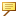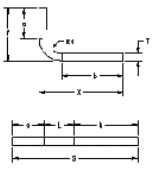Bend tables control bend allowance calculations of the developed length of flat material that is needed to make a bend in geometry with arcs. The developed length depends on the type of material, its thickness, and the bend radii. You can copy any number of bend tables to a part, but only one bend table can be assigned to a part at a time. All features associated with the assigned bend table are updated upon regeneration. You can also specify feature-specific bend allowance, by assigning a different bend table during the creation of a board segment.For bends with varying radii, such as cones or cylinders, use Y factor or K factor to calculate the developed length.
There are three standard bend tables for 90° bends available from the Machinery’s Handbook, 28th Edition:
Table
Material
Y factor
K factor
table 1
soft brass, copper
0.55
0.35
table 2
hard brass, copper, soft steel, aluminum
0.64
0.41
table 3
hard copper, bronze, cold rolled steel, spring steel
0.71
0.45
You can also create customized bend tables to support additional material types and methods for calculating developed length. If you create your own library of bend tables, set the file path using the configuration option material_upd_boardbend_table <full directory path>. The following information is included in a bend table:
Formula—Manages the bend allowance or developed length values with calculations and logic statements. The formula L = (Π/2 x R + Y factor x T) Θ/90 is defined by Creo Parametric and is used only for radius and thickness values outside the table data range.
Table Data—Lists radii and material thickness values with their corresponding bend allowance or developed length. A bend table must have at least one column and one row of tabulated data. You do not have to insert bend allowance data in every cell of the table. Any value not found in the table data is interpolated. If you only want to apply the bend table formulae, include data that is not used in your design (Radius = 1000, Thickness = 1000). Always specify table data for 90° bends. For bends other than 90°, the values are multiplied by Θ/90, where Θ is the specific bend angle, in degrees.
Materials Data—Lists the materials that the bend table can be applied. You receive a warning if your part's material type does not appear in this list. The materials must be listed between START MATERIALS and END MATERIALS. The materials are in the first column, uppercase, and one per line.The Materials Data list is case-sensitive. Make sure that the part's material type matches the one in the Materials Data list.
Conversion Equation—Uses bend allowance values from the bend table to calculate the developed length. For example, the conversion equation, L = 2 * (T + R)-A), makes adjustments to the bend allowance values as shown in the example below. When the specified thickness and bend radius values are within the table data range but not displayed in the table, the corresponding bend allowance is calculated by interpolation of the table bend allowance values. For details, refer to the interpolation method used for computing bend allowance discussed below. If no conversion equation is defined, then the developed length is equal to the bend allowance. If the radius and thickness values are outside the table data range, the bend table is ignored and the formula defined by Creo Parametric is used.
The following example illustrates an equation for specific bend angle ranges:
If ANGLE > 0 or ANGLE < = 90, a known parameter is used to compute the developed length.
SFLAT = X + Y - A
Known:
X = T + R + b
SFLAT = a + b + L
By Substitution:
a + b + L = (T + R + a) — A
or
The Conversion equation:
L = 2 * (T + R) — AWhere,
T = Thickness
ANGLE = Bend Angle
A = Bend Allowance
SFLAT = X + Y - A, where SFLAT is the total strip length
The known parameters that are used to calculate the developed length are:
X = T + R +b
Y = T + R + a
SFLAT = a + b + L
By substitution:
a + b + L = (T + R + b) + (T + R + a) - A
OR,
L = 2 * (T + R)-A which is the CONVERSION equation.
For example, the following program illustrates how a formula defined by Creo Parametric and a conversion equation are used for interpolation:
FORMULA
IF R<=2
IF ANGLE > 0 & ANGLE < 90
L = (ANGLE * PI/180) * (R + T/2)
L = (ANGLE * PI/180) * (R + T/2)
ENDIF
IF ANGLE >= 90 & ANGLE < 180
L = (ANGLE * PI/180) * (R + T/3)
ENDIF
ENDIF
IF R>2
L = (ANGLE * PI/180) * (R)
ENDIF
END FORMULA
!
CONVERSION
IF ANGLE > 0 & ANGLE <=90
L = 2 * (T + R) - .4285 * A
ELSE
L = 2 * (T + R) - .3567917 * A
ENDIF
END CONVERSION
The following is the equation for the Interpolation Method used for computing bend allowance:
A1,1*(Ty-T0)*(RY-R0) + A0,1*(T1-Ty)*(Ry-R0) + A1,0*(TY-T0)*(R1-RY) + A0,0*(T1-TY)*(R1-RY)
Ay = --------------------------------------------------------------------------------------------------
(T1-T0)*(R1-R0)
Where,
A0,0 is allowance for T0,R0
A1,0 is allowance for T1,R0
A0,1 is allowance for T0,R1
A1,1 is allowance for T1,R1
In the above example, T0 < Ty <T1 and R0 < Ry < R1.
When T0 = T1 = Ty, you can use the following formula:
A1,1 (Ry-R0) + A1,0 (R1-Ry)
Ay =  -----------------------------
(R1 - R0)
In the above example, A1,0 = A0,0 and A1,1 = A0,1.
When R0 = R1 = Ry, you can use the following formula:
A1,1 (Ty -T0) + A0,1 (T1-Ty)
Ay =  ------------------------------
(T1 -T0)
In the above example, A0,0 = A1,1 = A1,0
Where,
T = Thickness